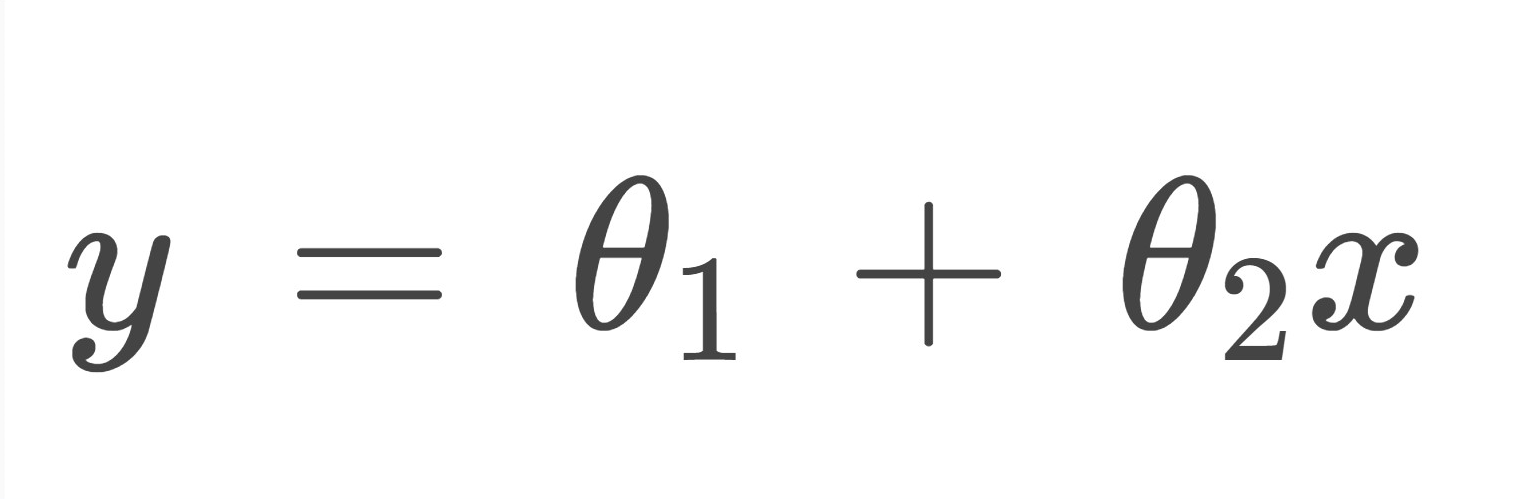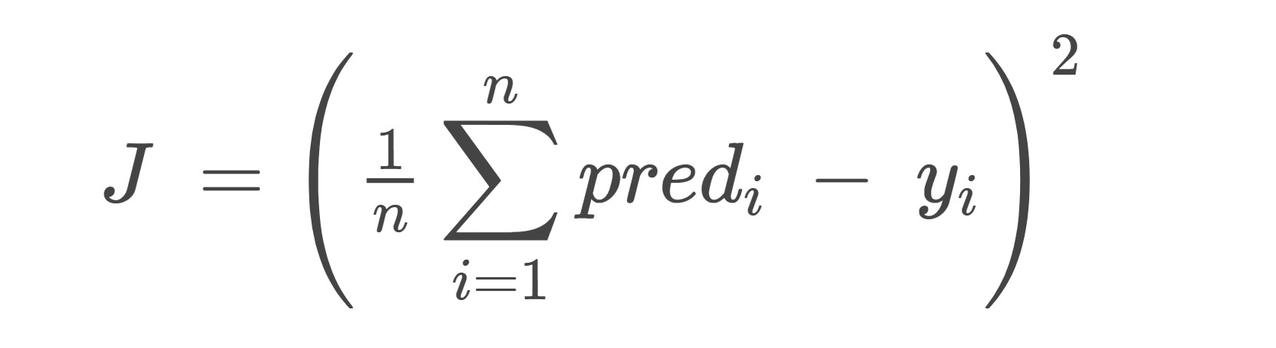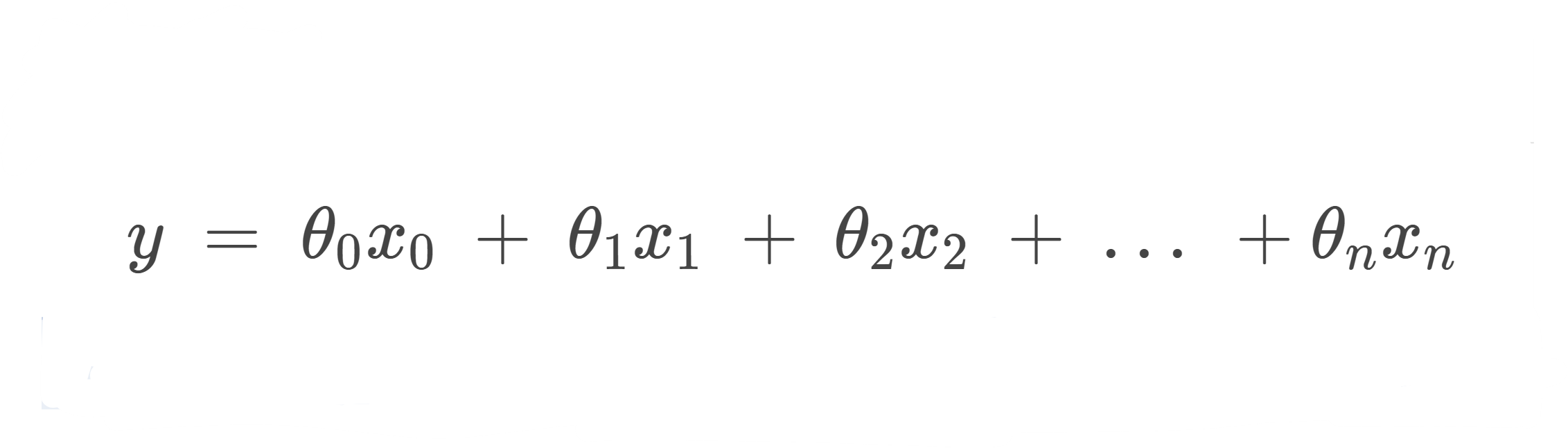featured

# Deep Dive – What is Linear Regression in Machine Learning?For nearly seventy years now, machine learning has had this crude definition attached to it: that it is a way to give computers and machines the ability to learn and apply knowledge; and while that definition is in no way wrong, there is so much involved in the process of learning and the process of applying the knowledge that it seems almost too simple to be true.
That’s not to say that it isn’t.

Even the simplest definitions of machine learning revolve around two major components: The learning part, and the applying knowledge part. Now, if I started explaining each and every method of both of those steps in this Deep Dive, we would have to spend the rest of the year here (and truth be told, I don’t know all of them). We can, however, start with one of the simplest methods of training (the learning part) and predicting (the applying knowledge part): Linear Regression.

Classification vs. Regression
To understand what Linear Regression is, we will first have to understand the two types of predictions that ML models make. In their simplest forms, Machine Learning models either predict a class to which a particular input value (known as an instance) belongs to or, they predict a quantity for an input value. The former is known as Classification while the latter is called Regression. So, in other words:

• Classification is the act of predicting a particular label (output class) for a particular instance and
• Regression is the act of predicting a continuous quantity for an input value.

An example of a Classification model is that of an image recognition software that recognises the animal present in the picture and sorts pictures out accordingly. A Regression example would be that of a height prediction model that takes in specific quantities and parameters related to a child’s health, genetics, their environment, and many others and in turn, predicts how tall they will be in a specific amount of time.

Definition
Now that we understand the difference between the two types of predictive models, lets Dive Deeper into what Linear Regression means.
By the very definitions of the two words involved, we can tell that Linear Regression will be a type of algorithm that gives us a continuous quantitative prediction involving a linear relationship between the inputs and the output values.

A Linear Regression model will essentially find a linear relationship between the inputs and the outputs and use the equation it generates from that relationship to predict the outputs of the incoming data instances.

Simple Linear Regression
A proper definition, given by towardsdatascience is that Simple Linear Regression is a, “type of regression analysis where the number of independent variables is one and there is a linear relationship between the independent (x) and dependent (y) variable.”

If you were, or still are a math enthusiast, you’ll remember that one of the main concepts involved in finding an equation of a straight line was a simple y = mx + c equation, where m was the gradient of the independent variable and c was the y-intercept; this method uses the same equation but with different variable symbols. In Linear Regression, we mostly use:Where x and y are the independent and dependant variables, respectively, θ1 is the y-intercept, and θ2 is the coefficient of x. Another thing to notice here is that there is only one independent variable in this equation, making it a Univariate Linear Regression equation.

But how do we find the two θ values?
Well, this is where this model gets a little tricky (not too much, though). The idea starts with two reasonably random θ values which get updated with each passing instance of training data. Because the main concept revolves around plotting a line of best fit onto a graph with x and y coordinates and using the line to predict future values, the line must be of least error, meaning the difference between the actual y values and y values predicted by the line, the Cost, must be minimised. The function, also known as the Cost Function (J), which is as follows:is to be minimised.

All this formula does is sum up the difference between the predicted y values and the actual y values, multiplies it by a normalising factor (1/n, in this case) and then square the whole thing. This is also known as the Root Mean Squared Error (RMSE) between the two different y values.

Are you still reading? Okay, good, bear with me for just a little while longer. The next main concept involved is that of minimising the value of this Cost function; essentially minimising the difference between the two y values. We do this by minimising the two constants, the two thetas mentioned above. The process, known as Gradient Descent, involves starting out with pseudorandom values for the two thetas and updating them with every passing iteration. This process involves a learning rate and iteratively, in moderately sized steps (determined by the learning rate), Gradient Descent reaches the minimum cost function.

Now, within Gradient Descent there can be two types, Batch Gradient Descent (BGD): a formula that considers all the gradients computed before computing the ith value and figuring out the new theta values; and Stochastic Gradient Descent (SGD): Taking in the gradient of one training sample at a time and updating the two thetas (the weights). BGD is used for relatively small models whereas SGD is used for larger models, where computing the values using all the previous gradients becomes an extremely time-consuming task. There is also a Mini version of BGD where a small batch of training data is taken and fed through SGD to compute the gradient for that Mini Batch.

And that’s basically it! A Linear Regression Model involves training a model to find values of the two thetas by minimising the Cost of a system and are used to compute values for the next instance to come. Although it’s pretty simple when using a Univariate System, it gets complicated and time consuming when Multiple independent variables get involved in a Multivariate Linear Regression Model. The concept, however, stays the same in that you have a theta value attached to every independent variable and the ultimate goal is to minimise the Cost of the function.Conclusion
Linear Regression, no matter how easy it sounds on paper, can become very time consuming and complicated. Linear Regression is used in predictive models with the ultimate goal of minimising the error in mind. Truth be told, every single ML models gets complicated because the predictions that these models make can never truly just be based off of a single independent variable. As programmers and practitioners, however, we just have to have a clear understanding of the real life model that we are trying to automate and profound knowledge of the type of model we are using.## ConsoliAds Sets Off As the First Mobile Ads Management Platform to Offer Monthly Payouts in Pakistan

in featured
·## Here are 10 key takeaways from renowned WordPress business owners

in featured
·in
·